# MP Board Class 11th Chemistry Important Questions States of Matter

## MP Board Class 11th Chemistry Important Questions Chapter 5 States of Matter

### States of Matter Important Questions

States of Matter Objective Type Questions

Question 1.
Choose the correct answer:

Question 1.
At the time of opening the bottle of ammonia, it can be recognized from a distance because:
(a) It is very reactive
(b) It diffuses very fast
(c) It possess a pungent smell
(d) It is lighter than air
Answer:
(b) It diffuses very fast

Question 2.
Who established the relationship between density and rate of diffusion of a gas:
(a) Boyle
(b) Charles
(c) Graham
(d) Avogadro
Answer:
(c) Graham

Question 3.
The value of in Calorie (approx):
(a) 1
(b) 2
(c) 3
(d) 4
Answer:
(b) 2Question 4.
Value of gas constant R is:
(a) 8314 × 107 ergs/degree/mol
(b) 83.14 × 106 ergs/degree/mol
(c) 83.14 × 105 ergs/degree/mol
(d) 8.314 × 107 ergs/degree/mol
Answer:
(d) 8.314 × 107 ergs/degree/mol

Question 5.
Absolute temperature is:
(a) 0° C
(b) – 100° C
(c) – 273° C
(d) – 373°C
Answer:
(c) – 273° C

Question 6.
To get general gas equation which two laws are combined:
(a) Charle’s law and Dalton’s law
(b) Graham’s law and Dalton’s law
(c) Boyle’s law and Charle’s law
(d) Avogadro’s law and Dalton’s law
Answer:
(c) Boyle’s law and Charle’s law

Question 7.
Which is correct in the following:
(a) r.m.s. velocity = 0.9212 × average velocity
(b) average velocity = 0.9212 × r.m.s. velocity
(c) r.m.s. velocity = 0.9013 × average velocity
(d) average velocity = 0.9013 × r.m.s. velocity
Answer:
(b) average velocity = 0.9212 × r.m.s. velocity

Question 8.
At constant volume the pressure of monoatomic gas depends on:
(a) Thickness of the wall of the vessel
(b) Absolute temperature
(c) Atomic number of the element
(d) Number of valence electron.
Answer:
(b) Absolute temperatureQuestion 9.
Behaviour of real gases near to that of ideal gases if:
(a) Temperature is low
(b) Pressure is high
(c) Low pressure and high temperature
(d) Gas is monoatomic
Answer:
(c) Low pressure and high temperature

Question 10.
At mountains of high altitude, water boils at lower temperature because of:
(a) Low atmospheric pressure
(b) High atmospheric pressure
(c) Hydrogen bonding in water is more strong at height
(d) Water vapour is lighter than liquid
Answer:
(a) Low atmospheric pressure

Question 11.
If molecular masses of two gases A and B are 16 and 64 respectively ratio of rates of diffusion A and B will be:
(a) 1:4
(b) 4:1
(c) 2:1
(d) 1:2
Answer:
(c) 2:1

Question 12.
Gases deviate from ideal behavior at high pressure because:
(a) At pressure number of colloision of molecule increases
(b) Attraction between molecules increases at high pressure
(c) Size of molecule decreases at high pressure.
(d) Molecule become steady at high pressure.
Answer:
(b) Attraction between molecules increases at high pressureQuestion 13.
Distance on which magnitude of energy is minimum is called:
(a) Atomic radius
(b) Lattice radius
(c) Critical distance
(d) Molecular distance
Answer:
(a) Atomic radius

Question 14.
Diffusion rate of methane is twice than that of gas x, molecular mass of gas x will be:
(a) 64
(b) 32
(c) 40
(d) 8.0
Answer:
(a) 64

Question 15.
Part of van der Waals’ equation which illustrate the inter molecule force of real gases:
(a) (V – b)
(b) RT
(c) [P+$$\frac{a}{VL}$$]
(d) (RT)-1
Answer:
(c) [P+$$\frac{a}{VL}$$]

Question 16.
Temperature which is same in both Celsius scale and Fahrenheit scale:
(a) 0°C
(b) 32°F
(c) – 40°C
(d) 40°C
Answer:
(c) – 40°CQuestion 2.
Fill in the blanks:

1. With increase in temperature viscocity ………………………..
2. S.I. unit of surface tension is ………………………….
3. ………………………… is more heavy among dry air and moist air
4. AT N.T.P., volume of gas equal to Avogrado’s numbers ………………………….
5. Unit of vander Waals’ constant ‘b’ is ………………………..
6. …………………………. has minimum value among average velocity, root mean square velocity and most probable velocity
7. Process of diffusion of air from the puncture of an autommobile tube is known as ……………………….
8. …………………………… is obtained if a graph is plotted between volume and absolute temperature
9. Apparatus used for measuring gas pressure is …………………………
10. S.I. unit of viscosity is ………………………….
11. Absolute temperature of ideal gas is ………………………… to the average kinetic theory of the molecule.
12. A liquid which is permanently super cooled is called ………………………..
13. According to kinetic theory, the average kinetic energy of a gas is directly proportional to its …………………………. temperature.
14. The kinetic energy of one mole of a gas is equal to …………………………..
15. Root mean square velocity is …………………………..

Answer:

1. Decreases
2. Nm-1
3. Dry air
4. 22.41
5. Litre/mol
6. Most probable velocity
7. Effiission
8. Straight line
9. Monometer
10. Nm-2S
11. Proportional
12. Glass
13. Absolute
14. $$\frac{3}{2}$$ RT
15. $$\sqrt { \frac { 3PV }{ M } }$$ or $$\sqrt { \frac { 3RT }{ M } }$$Question 3.
Answer in one word/sentence:

1. Vaporization of ethanol is faster as compared to water?
2. The resistance produced in the flow of a liquid is called?
3. Unit of surface tension is?
4. Molecular mass of two gases A and B are 36 and 64 respectively. What will be the ratio of diffusion of the two gases?
5. Write S.I. unit of pressure?
6. If rate of diffusion of oxygen is r, then tell the rate of diffusion of hydrogen?
7. Compressibility factor of ideal gases is?
8. Vander Waals’ equation is?
9. Adiabatic expression of ideal gas is?
10. Number of electron in outer most orbit of crypton is?

Answer:

1. Value of molar vaporization enthalpy of water is high
2. Viscosity
3. Dyne per cm
4. 4:3
5. pascal
6. r1 = 4r [$$\frac { r_{ 1 } }{ r_{ 2 } }$$ = $$\sqrt { \frac { m_{ 2 } }{ m_{ 1 } } }$$]
7. 1.0
8. [P + $$\frac { an^{ 2 } }{ V^{ 2 } }$$] [V – nb] = nRT
9. q = 0
10. 8 electron

Question 4.
Match the following:
[I]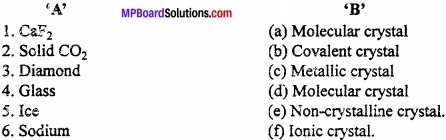Answer:

1. (f)
2. (a)
3. (b)
4. (a)
5. (d)
6. (c)

[II]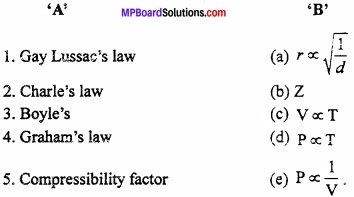Answer:

1. (d)
2. (c)
3. (e)
4. (a)
5. (b)

States of Matter Very Short Answer Type Questions

Question 1.
The resistance produced in the flow of a liquid is called?
Answer:
Viscosity.

Question 2.
Unit of surface tension is?
Answer:
Dyne per cm.

Question 3.
Write S.I. unit of pressure?
Answer:
Pascal.Question 4.
Name the scientist who developed relation between density and rate of diffusion of gas?
Answer:
Graham’s.

Question 5.
What is the Value of gas constant in S.I. unit?
Answer:
8.314 JK-1 mol-1

Question 6.
1 Pascal is equal to?
Answer:
1 Nm2.

Question 7.
Who established the relationship between density and rate of diffusion of gas?
Answer:
Graham’s.

Question 8.
Absolute temperature is?
Answer:
~273°C.

Question 9.
The value of R in calorie (approx)?
Answer:
2.Question 10.
Behaviour of real gases is near to that of ideal gases of?
Answer:
Low pressure and high temperature.

Question 11.
At mountains or high altitude, water boils at lower temperature because of?
Answer:
Low atmospheric pressure.

Question 12.
To get general gas equation. Which two laws are combined?
Answer:
Boyle’s law and Charle’s law.

Question 13.
At constant volume the pressure of gas depends upon?
Answer:
On absolute temperature.

Question 14.
Part of van der Waals’ equation which illustrate the inter – molecular forces of real gases?
Answer:
P + $$\frac { a }{ V^{ 2 } }$$.

Question 15.
Temperature which is same in both Celsius scale and fahrenheit scale?
Answer:
– 40°C.Question 16.
What is the formula for kinetic theory of gases?
Answer:
PV = $$\frac{1}{3}$$ mnv2.

Question 17.
The average kinetic energy of gases is proportional to?
Answer:
Absolute temperature.

Question 18.
What is the kinetic energy of 1 mole of gas?
Answer:
$$\frac{3}{2}$$ RT.

Question 19.
What is the formula of Root mean square velocity?
Answer:
$$\sqrt { \frac { 3PV }{ M } }$$ or $$\sqrt { \frac { 3RT }{ M } }$$.

Question 20.
Which type of Crystals are diamond and ice?
Answer:
Diamond – Covalent crystal
Ice – Ionic crystal.Question 21.
What is Critical temperature?
Answer:
The temperature above which the gas cannot be liquefied.

Question 22.
Poise is the unit of which basic property?
Answer:
Viscosity (1 poise = dynes/cm2s).

Question 23.
What is the unit of a (volume correction), b (pressure correction) in van der Waals’ equation?
Answer:
a (Volume correction) = atm L2 mol-2
b (Pressure correction) = L mol-1

States of Matter Short Answer Type Question – I

Question 1.
Explain Anisotropic and Isotropic?
Answer:
Anisotropic:
The crystalline solid exhibits different physical properties in the , three direction. In this way, crystalline solids are called anisotropic.

Isotropic:
The amorphous solid exhibits same physical properties in all directions. Due to this property they are called isotropic.

Question 2.
Define the term absolute zero? Write its value in centigrade scale?
Answer:
The lowest possible temperature at which all the gases are supposed to occupy zero volume is called absolute zero. The actual value of absolute zero is – 273.15°C. It is , related to temperature in centigrade scale by this relation.
t°C = t + 273 K.

Question 3.
What is unit cell?
Answer:
Smallest unit is a crystal which is formed by systematic arrangements of constituent particles as atom, molecule or ions, is called unit cell. The unit cell generates the whole lattice translation.Question 4.
What is crystal lattice?
Answer:
Geometry or shape of any crystal in which unit cells are arranged systematically and three dimensionally is called crystal lattice.

Question 5.
How does volume of balloon used for weather study, change with height?
Answer:
At height, atmospheric pressure decreases. The volume of gas inside the balloon increases with decrease in pressure. A stage comes when due to decrease in atmospheric pressure in larger extent, volume increases and balloon bursts.

Question 6.
In winter season, a layer of ice is formed in the lake but the fishes and other organisms present in the lake remain alive. Why?
Answer:
The maximum density of water is at 4°C but below 4°C temperature the density decreases. When the temperature of lake decreases then the water present on the surface become denser and goes downward. This occurs upto the level when the temperature rises to t 4°C. (MPBoardSolutions.com) If the temperature of the upper layer is less than 4°C, the water remains on the upper surface and converts into ice slowly and the water below the surface remains as such due to high density and remains as liquid. That is why, the fishes and micro – organisms remains alive.Question 7.
Why is the density of hot gas is less in comparison to cold gas?
Answer:
According to Charle’s law, volume of any gas of definite mass is directly proportional to absolute temperature. On increasing the temperature volume of gas also increases, but increase in volume results decrease in density.

Question 8.
Why one feel sluggish, breathlessness and headache at high altitude?
Answer:
At high altitude, the pressure is less and the corresponding volume of air is more, Thus, air becomes less dense at high altitude and the oxygen in air becomes insufficient for normal breathing. This causes what is known as altitude sickness.

Question 9.
What is Critical temperature?
Answer:
The temperature to which gas must be cooled before it can be liquefied by com-pression is known as critical temperature and is represented by Tc.
Example: Critical temperature of CO2 gas is 31.1°C or 304.1K.

Question 10.
What is critical pressure and critical volume?
Answer:
Critical pressure:
The minimum pressure required to liquefy the gas at its critical temperature is known as critical pressure and denoted by Pc.
Example: Critical pressure of CO2 is 72.8 atm.
Critical Volume: The volume occupied by 1 mole of gas at the critical temperature and critical pressure is known as critical volume and denoted by Vc.
Example: Critical volume of C02 gas is 94 cm3/mol.Question 11.
Why are tyres of automobile inflated to lesser pressure in summer than in winter?
Answer:
As the automobiles move the temp, of tyre increases due to friction against the road. Consequently the air inside the tyre expand thereby the pressure exerted by air against the wall of tyre also increases. (MPBoardSolutions.com) In summer, there is increase in temperature hence increase in pressure is much more. This may leads bursting at tyre. In order to check bursting of tyre, the tyre are inflated with looser amount of air than in winter.

Question 12.
What would be the S.I. unit for the quantity PV2T2/n?
Answer:
$$\frac { PV^{ 2 }T^{ 2 } }{ n }$$ = $$\frac { (Nm^{ -2 })(m^{ 3 })^{ 2 }(K)^{ 2 } }{ mol }$$ = Nm4K2mol-1.

Question 13.
Explain on the basis of Charle’s law that minimum possible temperature is – 273°C
Answer:
According to Charle’s law:
Vt = V0 [1 + $$\frac { t }{ 273 }$$]
At t = – 273°C Vt = V0 [ 1 – $$\frac { 273}{ 273 }$$] = 0
Therefore at – 273°C, the volume of gas becomes 0 and below this temperature the volume becomes – ve which is meaningless.

Question 14.
Why are liquid drops spherical?
Answer:
Small drops are spherical in shape:
Surface tension tries to decrease the surface area of a given liquid for a given volume. Therefore, drops of liquid are spherical because for a given volume sphere has minimum volume.Question 15.
What is Root Mean Square velocity and Average velocity?
Answer:
1. Root Mean Square velocity:
It is defined as root of, mean of, square of, velocity of large no. of molecules of same gas. It is denoted by V.
V = $$\sqrt { \frac { v_{ 1 }^{ 2 }+v_{ 2 }^{ 2 }+v_{ 3 }^{ 2 }…..v_{ n }^{ 2 } }{ n } }$$
2. Average velocity:
It is defined as average of, velocity of all the molecules present in gas. It is denoted by Va.
Va = $$\frac { v_{ 1 }+v_{ 2 }+v_{ 3 }…..v_{ n } }{ n }$$

Question 16.
Explain the difference between Evaporation and Boiling?
Answer:
Differences between Evaporation and Boiling:
Evaporation:

1. Evaporation decreases spontaneously and occur at all temperatures.
2. Evaporation is a process of the liquid surface.
3. Evaporation is a slow process.

Boiling:

1. Boling takes place only when the vapour pressure of this liquid becomes equal to atmospheric pressure.
2. Boling is a process of the entire liquid and occurs in the form of bubbles inside the liquid.
3. Boling is a fast process.

Question 17.
What do you mean by compressibility factor of gases?
Answer:
The ratio of observed volume and caluculated volume of a gas at a given temperature and pressure is known as compressibility factor. It is denoted by Z.
Thus,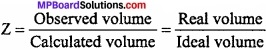or Z = $$\frac { PV }{ nRT }$$
For ideal gases, PV = nRrt
∴For ideal gas, Z = 1.

Question 18.
What is the effect of pressure on melting of ice?
Answer:
By increasing pressure there occur tremendous increase in kinetic energy of molecules, due to this at low temperature, the molecules move freely, hence on increasing pressure the ice below its melting point converted into liquid.

Question 19.
Mountaineers carry oxygen cylinders with them at the lance of climbing mountains. Why?
Answer:
Atmospheric pressure is relatively low at heights. Quantity of oxygen is low in mountain and climbers feel difficulties in breathing. Therefore, they carry oxygen cylinders along with them.Question 20.
Define viscosity of liquid. Explain the effect of temperature on viscosity?
Answer:
Resistance in flow of any liquid is called viscosity. Such resistance is produced due to internal friction of different layers of liquid. (MPBoardSolutions.com) When temperature is increased, the cohesive force, which opposes liquid flow, decreases and molecular velocity increases. Due to this, viscosity decreases.

Question 21.
On same temperature when ether and water pour on different hands, then ether seems to be more colder than water. Why?
Answer:
In ether the intermolecular attractive forces between the molecules is less in comparison to water, so ether evaporates more quickly than water and the energy required for evaporation is absorbed from hand, that is why ether seems to be colder.

Question 22.
What is surface tension? Write its S.L unit?
Answer:
It is an important property of a liquid which is related with interatomic attraction force. The molecules present inside the liquid is attracted equally by molecules present in all direction. (MPBoardSolutions.com)
But molecule present on the surface of liquid is attracted by molecules at bottom and in sides, as a result the molecules at surface are pulled downward and nature of surface is to lessen the area. Due to compactness, the surface of liquid behaves as a stretched membrane. This effect is called surface tension.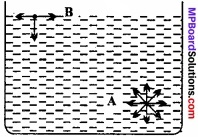“Surface tension is a measure of work which is necessary to increase the unit cross-section of liquid.”
Its S.I. unit is Joule/metre2 or Newton metre.

Question 23.
The compressibility factor Z of a gas is as follows:

1. What will be the value of Z for an ideal gas?
2. What will be the effect on Z above Boyle’s temperature for real gas?

Answer:

1. For ideal gas, compressibility factor Z = 1.
2. Above Boyle’s temperature, real gases show positive deviation. So, Z > 1.

Question 24.
What is Ideal gas? Write its characteristics?
Answer:
Ideal Gas:
The gases which obey Ideal gas equation under all conditions of temperature and pressure is called ideal gas.
Characteristics:

1. At constant temperature, product of pressure and volume of ideal gas are always constant. Therefore, horizontal line should be obtained in a graph. If graph is plotted between PV and P.
2. If an ideal gas is called at constant pressure, then its volume requestly decreased and become at – 273°C.
3. There is no force of attraction between gas molecules.
4. The compressibility factor of ideal gas is equal to one.Question 25.
What is Real gas? What are its properties?
Answer:
Gas which does not obey Boyle’s law, Charle’s law and Ideal gas equation strictly is called Real gas.
The gases which does not follow ideal gas equation behaviour under all condtions of temperature and pressure called real gas.
Properties:

1. They do not follow gas law at low temperature and high pressure.
2. At – 273°C their volume is not zero because most of the gases converted into liquid on cooling.
3. The attractive force between gas molecule is negligible.
4. The compressibility factor of real gas is not equal to zero.

States of Matter Short Answer Type Questions – II

Question 1.
State and explain Boyle’s Law?
Answer:
According to this law: “At constant temperature, the volume of a known amount of gas is inversely proportional to the pressure.”
P ∝ $$\frac{1}{V}$$ (at constant temperature)
⇒P = Constant × $$\frac{1}{V}$$
⇒ PV = Constant.
Thus, “at constant temperature, product of volume of a given mass of gas and pressure remain constant.”
At initial condition, P1V1 = K …………….. (1)
At final condition, P2V2 = K ………………. (2)
From eqn. (1) and eqn. (2).
P1V1 = P2V2

Question 2.
What is the nature of gas constant R?
Answer:
We know that,
PV = nRT
R = $$\frac{PV}{nT}$$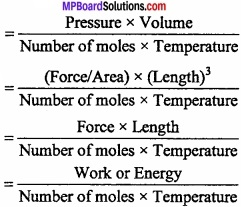Question 3.
Explain concept of absolute zero from Charle’s law?
Answer:
Charle’s law: According to this law “At a constant pressure the volume of certain mass of a gas increases or decreases by of its previous volume for every 1°C changes in temperature (increases or decreases).”
Suppose V0 is the volume of certain mass of gas at 0°C then,
Volume of the gas at 1°C temperature = V0 [1 + $$\frac{1}{273}$$]
Volume of the gas at t°C temperature = V0 [1 + $$\frac{t}{273}$$]
Volume of the gas at – 1°C temperature = V0 [1 – $$\frac{t}{273}$$]
Volume of gas at – 273°C = V0 [1 – $$\frac{273}{273}$$] = 0
Thus decrease of temperature results in the decrease in volume of the gas and ultimately the volume should become zero at – 273°C. (MPBoardSolutions.com) This lowest possible temperature at which all the gases are suppossed to occupy zero volume is called absolute zero.Question 4.
What is Gay Lussac’s law?
Answer:
Gay Lussac law:
According to this law, “At constant volume the pressure of a given mass of gas is directly proportional to its absolute temperature.”
P ∝ T (Mass and volume are constant)
⇒ P = K × T
⇒ $$\frac{P}{T}$$ = K
Suppose at initial condition,
$$\frac { P_{ 1 } }{ T_{ 1 } }$$ = K
At final condition,
$$\frac { P_{ 2 } }{ T_{ 2 } }$$ = K
From eqn. (1) and (2),
$$\frac { P_{ 1 } }{ T_{ 1 } }$$ = $$\frac { P_{ 2 } }{ T_{ 2 } }$$

Question 5.
State and explain Avogadro’s law?
Answer:
According to this law, “Equal volume of all gases under identical conditions of temperature and pressure contain equal number of molecules”.
V ∝N (at constant temperature and pressure) …………. (1)
At constant temperature and pressure number of moles of a gas n is directly proportional to number of molecules N.
Hence, N ∝n
⇒$$\frac{V}{n}$$ = Constant
Suppose at initial condition volume of gas is V1 and no. of mole of gas is n1 hence
$$\frac { V_{ 1 } }{ n_{ 1 } }$$ = Constant …………… (2)
Similarly at final condition no. of moles and volume of gas is n2 and V2, hence
$$\frac { V_{ 2 } }{ n_{ 2 } }$$ = Constant …………… (3)
From eqns. (2) and (3)
$$\frac { V_{ 1 } }{ n_{ 1 } }$$ = $$\frac { V_{ 2 } }{ n_{ 2 } }$$

Question 6.
Write the applications of Graham’s law of diffusion?
Answer:
1. To determine the density and molecular weight of a gas:
If the time of diffusion and density of a gas is known and the time of diffusion of other gas is known, then the density and molecular weight of other gas can be calculated.

2. Marsh gas indicator:
The persons working in the mines get aware by the leakage of the poisonous gases by this indicator.

3. In separation of gases:
The gases can be separated easily due to difference in the rate of diffusion of gases.

4. Smell:
Bad smell and poisonous gases get separated due to diffusion in air.Question 7.
Derive Charle’s law on the basis of Kinetic gas theory?
Answer:
According to Kinetic gas theory, kinetic energy of gases is directly proportional to absolute temperature.
K.E ∝T
$$\frac{1}{2}$$ mnv2 ∝ T
⇒$$\frac{1}{2}$$ mnv2 = KT
⇒$$\frac{3}{2}$$ × $$\frac{1}{3}$$ mnv2 = KT
⇒$$\frac{1}{3}$$ mnv2 = $$\frac{2}{3}$$ KT
⇒PV = $$\frac{2}{3}$$ KT,
⇒V = $$\frac{2}{3}$$ $$\frac{K}{P}$$.T
At constant pressure $$\frac{2}{3}$$ $$\frac{K}{P}$$ = constant
V = constant ∝ T
V ∝ T

Question 8.
How are rates of diffusion of different gases compared?
Answer:
Let two gases are A and B, equal volume V of both gases diffuse in times tA and tB respectively.
Rate of diffusion of gas A,
r A = $$\frac { V }{ t_{ A } }$$
Rate of diffusion of gas B,
r B = $$\frac { V }{ t_{ B } }$$
∴$$\frac { r_{ A } }{ r_{ B } }$$ = $$\sqrt { \frac { d_{ B } }{ d_{ A } } }$$
From equation (3) and equation (4),
$$\frac { t_{ B } }{ t_{ A } }$$ = $$\sqrt { \frac { d_{ B } }{ d_{ A } } }$$.Question 9.
The ratio between the rate of diffusion of an unknown gas (x) s&d CO2 Is 40:45 Find out the molecular mass of unknown gas (x).
Solution:
According to Graham’s law of diffusion,
$$\frac { r_{ 1 } }{ r_{ 2 } }$$ = $$\sqrt { \frac { M_{ 2 } }{ M_{ 1 } } }$$
Given, r1:r2 = 40:45 M2(CO2) = 44, M1 = ?
⇒$$\frac{40}{45}$$ = $$\sqrt { \frac { 44 }{ M_{ 1 } } }$$
⇒ $$\frac { (40)^{ 2 } }{ (45)^{ 2 } }$$ = $$\sqrt { \frac { 44 }{ M_{ 1 } } }$$
M1 = $$\frac { 44\times 45\times 45 }{ 40\times 40 }$$ = 55.68.

Question 10.
If relative density of chlorine is 36, diffusion of 25 volume of hydrogen takes 40 sec. under the condition how much time will be taken for the diffusion of 30 volume of chlorine?
Solution:
Hydrogen d1 = 1, r1 = $$\frac{25}{40}$$, Chlorine d2 = 36, r2 = $$\frac{30}{t}$$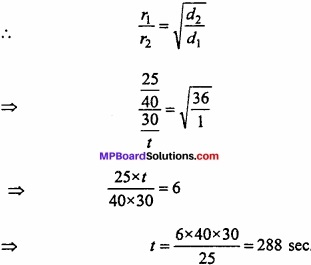States of Matter Long Answer Type Questions

Question 1.
State and explain Graham’s law of diffusion?
Answer:
Graham’s Law of Diffusion:
The rate of diffusion of gas under similar condition of temperature and pressure is inversely proportional to the square roots of their density.”
Thus, Rate of diffusion ∝$$\frac { 1 }{ \sqrt { density } }$$
⇒r ∝$$\frac { 1 }{ \sqrt { d } }$$
If the rate of diffusion of two gases are r1 and r2 and their density are d1 and d2 respectively.
r1 = K $$\frac { 1 }{ \sqrt { d_{ 1 } } }$$
r1 = K $$\frac { 1 }{ \sqrt { d_{ 2 } } }$$
$$\frac { r_{ 1 } }{ r_{ 2 } }$$ = $$\sqrt { \frac { d_{ 2 } }{ d_{ 1 } } }$$
∵ M. Mass = 2 × Vapour density = $$\frac { Molecular\quad mass }{ 2 }$$ = Vapour density
$$\frac { r_{ 1 } }{ r_{ 2 } }$$ = $$\sqrt { \frac { d_{ 2 } }{ d_{ 1 } } }$$ = $$\sqrt { \frac { M_{ 2 } }{ M_{ 1 } } }$$.Question 2.
Write difference between Real and Ideal gas?
Answer:
Differences between Ideal gas and Real gas:
Ideal gas:

1. Ideal gas obeys die equation, PV = RT at all temperature and pressure.
2. There is no ideal gas, they are hypothetical.
3. Total volume of gas molecules is supposed to be negligible in comparison to total volume.
4. There is no attraction force between gas molecules.

Real gas:

1. Real gas obeys PV = RT only at low pressure and high temperature.
2. All existing gases are real gases and show deviation from ideal gas behaviour, positive or negative.
3. Volume of gas molecules are not negligible in comparison to total volume.
4. Gas molecules attract each other.Therefore, total pressure is less than ideal gas.

Question 3.
What are the main differences between Crystalline and Amorphous solids?
Answer:
Differences between Crystalline and Amorphous solids:
Crystalline:

1. In it the constituent particles are arranged in regular manner.
2. Melting point of crystalline solid is fixed.
3. They are an anisotropic i.e. some of their physical properties are different in different directions.
4. They are rigid and their shape is not distorted by mild distorting tone.
5. They have a definite heat of fusion.
6. They are true solid in real meaning.

Amorphous solids:

1. In it the constituent particles are arranged in irregular manner.
2. Melting point of amorphous solid is not fixed.
3. They are Isotropic i.e. their physical properties are same in all directions.
4. They are not very rigid, they can be distorted easily.
5. They do not have definite heat of fusion.
6. They are super cooled liquid.Question 4.
Using state equation clarify that the density of a gas at given temperature is proportional to pressure of gas?
Answer:
PV = nRT
PV = $$\frac{m}{M}$$ RT,
Or P = $$\frac{mRT}{VM}$$,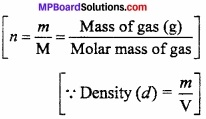Or P = $$\frac{dRT}{M}$$
Or d = $$\frac{PM}{RT}$$
if T = known constant
∴d ∝P

Question 5.
Derive Ideal gas equation on the basis of kinetic gas equation?
Answer:
According to Kinetic gas theory, “ The average kinetic energy of gas molecules is directly proportional to absolute temperature.”
Average Kinetic energy = $$\frac{1}{2}$$ mnv2
$$\frac{1}{2}$$ mnv2 ∝ T
⇒$$\frac{1}{2}$$ mnv2 = KT
⇒$$\frac{3}{2}$$ × $$\frac{1}{3}$$ mnv2 = KT
⇒$$\frac{1}{3}$$ mnv2 = $$\frac{2}{3}$$ KT,
⇒$$\frac{PV}{T}$$ = R
⇒ PV = RT.Question 6.
Explanation of Dalton’s law on the basis of Kinetic gas theory?
Answer:
If the volume of container V litre and no. of moles of gas A is n1 ar.d mass of each particles in m1 R.M.S. velocity is V1 then,
PA = $$\frac{1}{3}$$ $$\frac { m_{ 1 }n_{ 1 }v_{ 1 }^{ 2 } }{ V }$$
Kinetic gas equation PV = $$\frac{1}{3}$$ mnv2
For B gas number of moles = n2
Mass = m2
R.M.S. Velocity = v2
PB = $$\frac{1}{3}$$ $$\frac { m_{ 2 }n_{ 2 }v_{ 2 }^{ 2 } }{ V }$$
If both the gases are kept in same container total pressure of mixture
P = $$\frac{1}{3}$$ $$\frac { m_{ 1 }n_{ 1 }v_{ 1 }^{ 2 } }{ V }$$ + $$\frac{1}{3}$$ $$\frac { m_{ 2 }n_{ 2 }v_{ 2 }^{ 2 } }{ V }$$
⇒ P = PA + PB
so in general, for more than two gases
P = PA + PB + PC + …………………..
It is Daltons law of partial pressure.